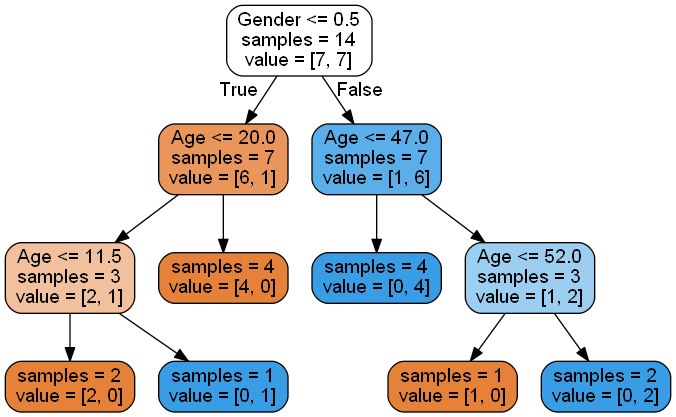• No products in the cart.

# 204.3.9 The Problem of Overfitting the Decision Tree

##### Exploring the overfitting of a Decision Tree.

Link to the previous post : https://statinfer.com/204-3-8-practice-validating-the-tree/

So far we have built a tree, predicted with our model and validated the tree. In this post we will handle the issue of over fitting a tree.

First, we will built another tree and see the problem of overfitting and then will find how to solve the problem.

### Practice : The Problem of Overfitting

• Import both test and training data
• Build a decision tree model on training data
• Find the accuracy on training data
• Find the predictions for test data
• What is the model prediction accuracy on test data?

### Solution

• Import both test and training data
```import pandas as pd

train.shape
```
`(14, 3)`
```test.shape
```
`(6, 3)`
```train.info()
```
```<class 'pandas.core.frame.DataFrame'>
RangeIndex: 14 entries, 0 to 13
Data columns (total 3 columns):
Age       14 non-null int64
Gender    14 non-null object
Bought    14 non-null object
dtypes: int64(1), object(2)
memory usage: 416.0+ bytes
```
```# the data have string values we need to convert them into numerica values
train['Gender'] = train['Gender'].map( {'Male': 1, 'Female': 0} ).astype(int)
train['Bought'] = train['Bought'].map({'Yes':1, 'No':0}).astype(int)

test['Gender'] = test['Gender'].map( {'Male': 1, 'Female': 0} ).astype(int)
test['Bought'] = test['Bought'].map({'Yes':1, 'No':0}).astype(int)
```
```train.info()
```
```<class 'pandas.core.frame.DataFrame'>
RangeIndex: 14 entries, 0 to 13
Data columns (total 3 columns):
Age       14 non-null int64
Gender    14 non-null int32
Bought    14 non-null int32
dtypes: int32(2), int64(1)
memory usage: 304.0 bytes
```
```from sklearn import tree

#Defining Features and lables
features = list(train.columns[:2])

X_train = train[features]
y_train = train['Bought']

#X_train

X_test = test[features]
y_test = test['Bought']

#training Tree Model
clf = tree.DecisionTreeClassifier()
clf.fit(X_train,y_train)
```
```DecisionTreeClassifier(class_weight=None, criterion='gini', max_depth=None,
max_features=None, max_leaf_nodes=None, min_samples_leaf=1,
min_samples_split=2, min_weight_fraction_leaf=0.0,
presort=False, random_state=None, splitter='best')```
```#Plotting the trees

dot_data = StringIO()
tree.export_graphviz(clf,
out_file = dot_data,
feature_names = features,
filled=True, rounded=True,
impurity=False)

graph = pydotplus.graph_from_dot_data(dot_data.getvalue())
Image(graph.create_png())
``````predict1 = clf.predict(X_train)
print(predict1)
```
```[1 1 1 0 0 1 1 1 0 0 0 1 0 0]
```
```predict2 = clf.predict(X_test)
print(predict2)
```
```[0 0 0 1 1 1]
```
```####Calculation of Accuracy and Confusion Matrix on the training data

from sklearn.metrics import confusion_matrix ###for using confusion matrix###
cm1 = confusion_matrix(y_train,predict1)
cm1
```
```array([[7, 0],
[0, 7]])```
```total1 = sum(sum(cm1))
#####from confusion matrix calculate accuracy
accuracy1 = (cm1[0,0]+cm1[1,1])/total1
accuracy1
```
`1.0`
```#Accuracy On Test Data
cm2 = confusion_matrix(y_test,predict2)
cm2
```
```array([[0, 2],
[3, 1]])```
```total2 = sum(sum(cm2))
#####from confusion matrix calculate accuracy
accuracy2 = (cm2[0,0]+cm2[1,1])/total2
accuracy2
```
`0.16666666666666666`

### The Problem of Overfitting

• If we further grow the tree we might even see each row of the input data table as the final rules.
• The model will be really good on the training data but it will fail to validate on the test data.
• Growing the tree beyond a certain level of complexity leads to overfitting.
• A really big tree is very likely to suffer from overfitting.

The next post is about pruning a decision tree in python.

Link to the next post : https://statinfer.com/204-3-10-pruning-a-decision-tree-in-python/